## A 0.110 kg cube of ice (frozen water) is floating in glycerine. The glycerine is in a tall cylinder that has inside radius 3.70 cm. The leve

Question

A 0.110 kg cube of ice (frozen water) is floating in glycerine. The glycerine is in a tall cylinder that has inside radius 3.70 cm. The level of the glycerine is well below the top cylinder. If the ice completely melts, by what distance does the height of the liquid in the cylinder change? Express your answer with the appropriate units. Enter positive value if the surface of the water is above the original level of the glycerin before the ice melted and negative value if the surface of the water is below the original level of the glycerin.

in progress 0
6 months 2021-07-20T03:34:00+00:00 1 Answers 40 views 0

the distance by which the height of the  liquid in the cylinder change after the ice gets melted = 0.528 cm

Explanation:

The change in volume of glycerin when the ice cube is placed on the surface of the glycerin can be represented as: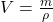Given that ;

the mass of the ice cube (m) = 0.11 kg = 0.110 × 10³ g

density of the glycerine (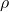) = 1.260 kg/L = 1.260 g/cm³

Then:

V =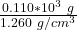V =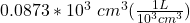V = 0.0873 L

Now;Initially the volume of the glycerin before the ice cube starts to melt is: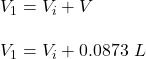However; the volume of the water produced by the 0.11 kg ice cube =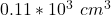The expression for change in the volume of glycerin after the ice cube starts to melt is as follows: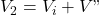replacing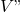with; we have: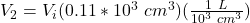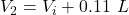The overall total change in the volume of the glycerin is illustrated as: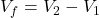Now; from the foregoing ; lets replace the respective value of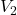and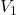in the above equation ; we have;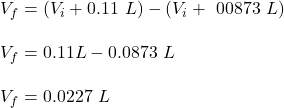The formula usually known to be the volume of a cylinder is :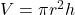For the question ; we will have: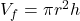making h the subject of the  formula ; we have: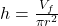replacing 0.0227 L for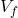and the given value of radius which is = 3.70 cm; we have: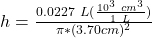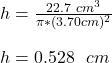Thus ; the distance by which the height of the  liquid in the cylinder change after the ice gets melted = 0.528 cm